# C.1   Source of definitions

The definitions of the basic statistical terms given in this annex are taken from International Standard ISO 3534‑1:1993* . This should be the first source consulted for the definitions of terms not included here. Some of these terms and their underlying concepts are elaborated upon in C.3 following the presentation of their formal definitions in C.2 in order to facilitate further the use of this Guide. However, C.3, which also includes the definitions of some related terms, is not based directly on ISO 3534‑1:1993.

# C.2   Definitions

As in Clause 2 and Annex B, the use of parentheses around certain words of some terms means that the words may be omitted if this is unlikely to cause confusion.

Terms C.2.1 to C.2.14 are defined in terms of the properties of populations. The definitions of terms C.2.15 to C.2.31 are related to a set of observations (see Reference ).

C.2.1
probability
a real number in the scale 0 to 1 attached to a random event

NOTE   It can be related to a long‑run relative frequency of occurrence or to a degree of belief that an event will occur. For a high degree of belief, the probability is near 1.

[ISO 3534‑1:1993, definition 1.1]
C.2.2
random variable
variate
a variable that may take any of the values of a specified set of values and with which is associated a probability distribution [ISO 3534‑1:1993, definition 1.3 (C.2.3)]

NOTE 1   A random variable that may take only isolated values is said to be “discrete”. A random variable which may take any value within a finite or infinite interval is said to be “continuous”.

NOTE 2   The probability of an event A is denoted by Pr(A) or P(A).

[ISO 3534‑1:1993, definition 1.2]

Guide Comment: The symbol Pr(A) is used in this Guide in place of the symbol Pr(A) used in ISO 3534‑1:1993.

C.2.3
probability distribution (of a random variable)
a function giving the probability that a random variable takes any given value or belongs to a given set of values

NOTE   The probability on the whole set of values of the random variable equals 1.

[ISO 3534‑1:1993, definition 1.3]
C.2.4
distribution function
a function giving, for every value x, the probability that the random variable X be less than or equal to x: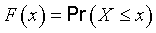[ISO 3534‑1:1993, definition 1.4]
C.2.5
probability density function (for a continuous random variable)
the derivative (when it exists) of the distribution function: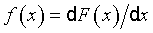NOTE   f(x) dx is the “probability element”:[ISO 3534‑1:1993, definition 1.5]
C.2.6
probability mass function
a function giving, for each value xi of a discrete random variable X, the probability pi that the random variable equals xi: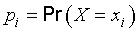[ISO 3534‑1:1993, definition 1.6]
C.2.7
parameter
a quantity used in describing the probability distribution of a random variable

[ISO 3534‑1:1993, definition 1.12]
C.2.8
correlation
the relationship between two or several random variables within a distribution of two or more random variables

NOTE   Most statistical measures of correlation measure only the degree of linear relationship.

[ISO 3534‑1:1993, definition 1.13]
C.2.9
expectation (of a random variable or of a probability distribution)
expected value
mean
1. For a discrete random variable X taking the values xi with the probabilities pi, the expectation, if it exists, is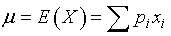the sum being extended over all the values xi which can be taken by X.
2. For a continuous random variable X having the probability density function f(x), the expectation, if it exists, is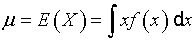the integral being extended over the interval(s) of variation of X.

[ISO 3534‑1:1993, definition 1.18]
C.2.10
centred random variable
a random variable the expectation of which equals zero

NOTE   If the random variable X has an expectation equal to μ, the corresponding centred random variable is (X − μ).

[ISO 3534‑1:1993, definition 1.21]
C.2.11
variance (of a random variable or of a probability distribution)
the expectation of the square of the centred random variable [ISO 3534‑1:1993, definition 1.21 (C.2.10)]: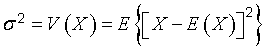[ISO 3534‑1:1993, definition 1.22]
C.2.12
standard deviation (of a random variable or of a probability distribution)
the positive square root of the variance:[ISO 3534‑1:1993, definition 1.23]
C.2.13
central moment2) of order q
in a univariate distribution, the expectation of the qth power of the centred random variable (X − μ):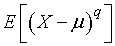NOTE   The central moment of order 2 is the variance [ISO 3534‑1:1993, definition 1.22 (C.2.11)] of the random variable X.

[ISO 3534‑1:1993, definition 1.28]
C.2.14
normal distribution
Laplace‑Gauss distribution
the probability distribution of a continuous random variable X, the probability density function of which isfor  < x < +.

NOTE   μ is the expectation and σ is the standard deviation of the normal distribution.

[ISO 3534‑1:1993, definition 1.37]
C.2.15
characteristic
a property which helps to identify or differentiate between items of a given population

NOTE   The characteristic may be either quantitative (by variables) or qualitative (by attributes).

[ISO 3534‑1:1993, definition 2.2]
C.2.16
population
the totality of items under consideration

NOTE   In the case of a random variable, the probability distribution [ISO 3534‑1:1993, definition 1.3 (C.2.3)] is considered to define the population of that variable.

[ISO 3534‑1:1993, definition 2.3]
C.2.17
frequency
the number of occurrences of a given type of event or the number of observations falling into a specified class

[ISO 3534‑1:1993, definition 2.11]
C.2.18
frequency distribution
the empirical relationship between the values of a characteristic and their frequencies or their relative frequencies

NOTE   The distribution may be graphically presented as a histogram (ISO 3534‑1:1993, definition 2.17), bar chart (ISO 3534‑1:1993, definition 2.18), cumulative frequency polygon (ISO 3534‑1:1993, definition 2.19), or as a two‑way table (ISO 3534‑1:1993, definition 2.22).

[ISO 3534‑1:1993, definition 2.15]
C.2.19
arithmetic mean
average
the sum of values divided by the number of values

NOTE 1   The term “mean” is used generally when referring to a population parameter and the term “average” when referring to the result of a calculation on the data obtained in a sample.

NOTE 2   The average of a simple random sample taken from a population is an unbiased estimator of the mean of this population. However, other estimators, such as the geometric or harmonic mean, or the median or mode, are sometimes used.

[ISO 3534‑1:1993, definition 2.26]
C.2.20
variance
a measure of dispersion, which is the sum of the squared deviations of observations from their average divided by one less than the number of observations

EXAMPLE   For n observations x1x2, ..., xn with average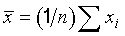the variance isNOTE 1   The sample variance is an unbiased estimator of the population variance.

NOTE 2   The variance is n(n − 1) times the central moment of order 2 (see note to ISO 3534‑1:1993, definition 2.39).

[ISO 3534‑1:1993, definition 2.33]

Guide Comment: The variance defined here is more appropriately designated the “sample estimate of the population variance”. The variance of a sample is usually defined to be the central moment of order 2 of the sample (see C.2.13 and C.2.22).

C.2.21
standard deviation
the positive square root of the variance

NOTE   The sample standard deviation is a biased estimator of the population standard deviation.

[ISO 3534‑1:1993, definition 2.34]
C.2.22
central moment of order q
in a distribution of a single characteristic, the arithmetic mean of the qth power of the difference between the observed values and their average x‾‾: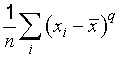where n is the number of observations

NOTE   The central moment of order 1 is equal to zero.

[ISO 3534‑1:1993, definition 2.37]
C.2.23
statistic
a function of the sample random variables

NOTE   A statistic, as a function of random variables, is also a random variable and as such it assumes different values from sample to sample. The value of the statistic obtained by using the observed values in this function may be used in a statistical test or as an estimate of a population parameter, such as a mean or a standard deviation.

[ISO 3534‑1:1993, definition 2.45]
C.2.24
estimation
the operation of assigning, from the observations in a sample, numerical values to the parameters of a distribution chosen as the statistical model of the population from which this sample is taken

NOTE   A result of this operation may be expressed as a single value [point estimate; see ISO 3534‑1:1993, definition 2.51 (C.2.26)] or as an interval estimate [see ISO 3534‑1:1993, definitions 2.57 (C.2.27) and 2.58 (C.2.28)].

[ISO 3534‑1:1993, definition 2.49]
C.2.25
estimator
a statistic used to estimate a population parameter

[ISO 3534‑1:1993, definition 2.50]
C.2.26
estimate
the value of an estimator obtained as a result of an estimation

[ISO 3534‑1:1993, definition 2.51]
C.2.27
two‑sided confidence interval
when T1 and T2 are two functions of the observed values such that, θ being a population parameter to be estimated, the probability Pr(T1 ≤ θ ≤ T2) is at least equal to (1 − α) [where (1 − α) is a fixed number, positive and less than 1], the interval between T1 and T2 is a two‑sided (1 − α) confidence interval for θ

NOTE 1   The limits T1 and T2 of the confidence interval are statistics [ISO 3534‑1:1993, definition 2.45 (C.2.23)] and as such will generally assume different values from sample to sample.

NOTE 2   In a long series of samples, the relative frequency of cases where the true value of the population parameter θ is covered by the confidence interval is greater than or equal to (1 − α).

[ISO 3534‑1:1993, definition 2.57]
C.2.28
one‑sided confidence interval
when T is a function of the observed values such that, θ being a population parameter to be estimated, the probability Pr(T ≥ θ) [or the probability Pr(T ≤ θ)] is at least equal to (1 − α) [where (1 − α) is a fixed number, positive and less than 1], the interval from the smallest possible value of θ up to T (or the interval from T up to the largest possible value of θ) is a one‑sided (1 − α) confidence interval for θ

NOTE 1   The limit T of the confidence interval is a statistic [ISO 3534‑1:1993, definition 2.45 (C.2.23)] and as such will generally assume different values from sample to sample.

NOTE 2   See Note 2 of ISO 3534‑1:1993, definition 2.57 (C.2.27).

[ISO 3534‑1:1993, definition 2.58]
C.2.29
confidence coefficient
confidence level
the value (1 − α) of the probability associated with a confidence interval or a statistical coverage interval. [See ISO 3534‑1:1993, definitions 2.57 (C.2.27), 2.58 (C.2.28) and 2.61 (C.2.30).]

NOTE    (1 − α) is often expressed as a percentage.

[ISO 3534‑1:1993, definition 2.59]
C.2.30
statistical coverage interval
an interval for which it can be stated with a given level of confidence that it contains at least a specified proportion of the population

NOTE 1   When both limits are defined by statistics, the interval is two‑sided. When one of the two limits is not finite or consists of the boundary of the variable, the interval is one‑sided.

NOTE 2   Also called “statistical tolerance interval”. This term should not be used because it may cause confusion with “tolerance interval” which is defined in ISO 3534‑2:1993.

[ISO 3534‑1:1993, definition 2.61]
C.2.31
degrees of freedom
in general, the number of terms in a sum minus the number of constraints on the terms of the sum

[ISO 3534‑1:1993, definition 2.85]

# C.3   Elaboration of terms and concepts

## C.3.1   Expectation

The expectation of a function g(z) over a probability density function p(z) of the random variable z is defined by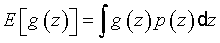where, from the definition of p(z), p(z)dz = 1. The expectation of the random variable z, denoted by μz, and which is also termed the expected value or the mean of z, is given byIt is estimated statistically by z‾‾, the arithmetic mean or average of n independent observations zi of the random variable z, the probability density function of which is p(z):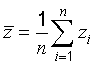## C.3.2   Variance

The variance of a random variable is the expectation of its quadratic deviation about its expectation. Thus the variance of random variable z with probability density function p(z) is given by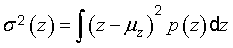where μz is the expectation of z. The variance σ2(z) may be estimated by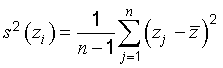whereand the zi are n independent observations of z.

NOTE 1   The factor n − 1 in the expression for s2(zi) arises from the correlation between zi and z‾‾ and reflects the fact that there are only n − 1 independent items in the set {zi − z‾‾}.

NOTE 2   If the expectation μz of z is known, the variance may be estimated by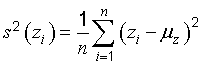The variance of the arithmetic mean or average of the observations, rather than the variance of the individual observations, is the proper measure of the uncertainty of a measurement result. The variance of a variable z should be carefully distinguished from the variance of the mean z‾‾. The variance of the arithmetic mean of a series of n independent observations zi of z is given by σ2(z‾‾) = σ2(zi)n and is estimated by the experimental variance of the mean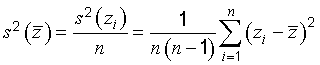## C.3.3   Standard deviation

The standard deviation is the positive square root of the variance. Whereas a Type A standard uncertainty is obtained by taking the square root of the statistically evaluated variance, it is often more convenient when determining a Type B standard uncertainty to evaluate a nonstatistical equivalent standard deviation first and then to obtain the equivalent variance by squaring the standard deviation.

## C.3.4   Covariance

The covariance of two random variables is a measure of their mutual dependence. The covariance of random variables y and z is defined by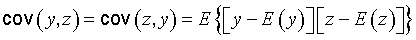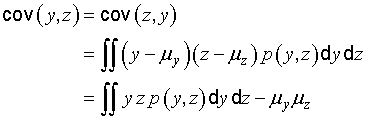where p(yz) is the joint probability density function of the two variables y and z. The covariance cov(yz) [also denoted by υ(yz)] may be estimated by s(yizi) obtained from n independent pairs of simultaneous observations yi and zi of y and z,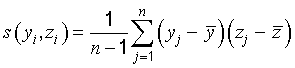whereandNOTE   The estimated covariance of the two means y and z is given by s(yz ) = s(yizi)n.

## C.3.5   Covariance matrix

For a multivariate probability distribution, the matrix V with elements equal to the variances and covariances of the variables is termed the covariance matrix. The diagonal elements, υ(zz) ≡ σ2(z) or s(zizi) ≡ s2(zi), are the variances, while the off‑diagonal elements, υ(yz) or s(yizi), are the covariances.

## C.3.6   Correlation coefficient

The correlation coefficient is a measure of the relative mutual dependence of two variables, equal to the ratio of their covariances to the positive square root of the product of their variances. Thuswith estimatesThe correlation coefficient is a pure number such that −1 ≤ ρ ≤ +1 or −1 ≤ r(yizi) ≤ +1.

NOTE 1   Because ρ and r are pure numbers in the range −1 to +1 inclusive, while covariances are usually quantities with inconvenient physical dimensions and magnitudes, correlation coefficients are generally more useful than covariances.

NOTE 2   For multivariate probability distributions, the correlation coefficient matrix is usually given in place of the covariance matrix. Since ρ(yy) = 1 and r(yiyi) = 1, the diagonal elements of this matrix are unity.

NOTE 3   If the input estimates xi and xj are correlated (see 5.2.2) and if a change δi in xi produces a change δj in xj, then the correlation coefficient associated with xi and xj is estimated approximately byThis relation can serve as a basis for estimating correlation coefficients experimentally. It can also be used to calculate the approximate change in one input estimate due to a change in another if their correlation coefficient is known.

## C.3.7   Independence

Two random variables are statistically independent if their joint probability distribution is the product of their individual probability distributions.

NOTE   If two random variables are independent, their covariance and correlation coefficient are zero, but the converse is not necessarily true.

## C.3.8   The t‑distribution; Student's distribution

The t‑distribution or Student's distribution is the probability distribution of a continuous random variable t whose probability density function is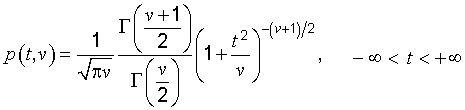where Γ is the gamma function and v > 0. The expectation of the t‑distribution is zero and its variance is v/(v − 2) for v > 2. As v → , the t‑distribution approaches a normal distribution with μ = 0 and σ = 1 (see C.2.14).

The probability distribution of the variable (z‾‾ − μz)s(z‾‾) is the t‑distribution if the random variable z is normally distributed with expectation μz, where z is the arithmetic mean of n independent observations zi of z, s(zi) is the experimental standard deviation of the n observations, and s(z‾‾) = s(zi)n‾‾‾ is the experimental standard deviation of the mean z‾‾ with v = n − 1 degrees of freedom.

2)   If, in the definition of the moments, the quantities X, X − a, Y, Y − b, etc. are replaced by their absolute values, i.e. X, X − a, Y, Y − b, etc., other moments called “absolute moments” are defined.

*   Footnote to the 2008 version:
ISO 3534‑1:1993 has been cancelled and replaced by ISO 3534‑1:2006. Note that some of the terms and definitions have been revised. For further information, see the latest edition.